# IB DP Physics: HL复习笔记7.3.1 Discovery of the Nucleus

### The Rutherford-Geiger-Marsden Experiment

• Evidence for the structure of the atom was discovered by Ernest Rutherford in the beginning of the 20th century from the study of α-particle scattering
• The experimental setup consists of alpha particles fired at thin gold foil and a detector on the other side to detect how many particles deflected at different angles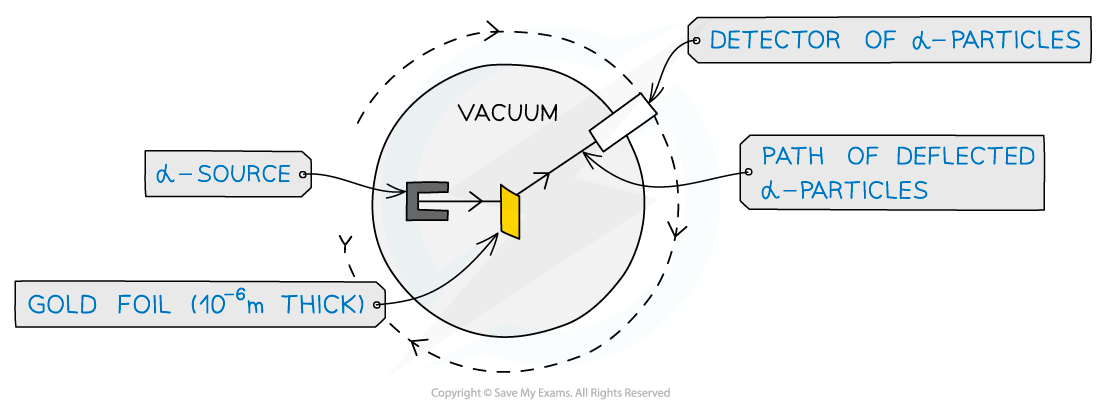α-particle scattering experiment set up

• α-particles are the nucleus of a helium atom and are positively charged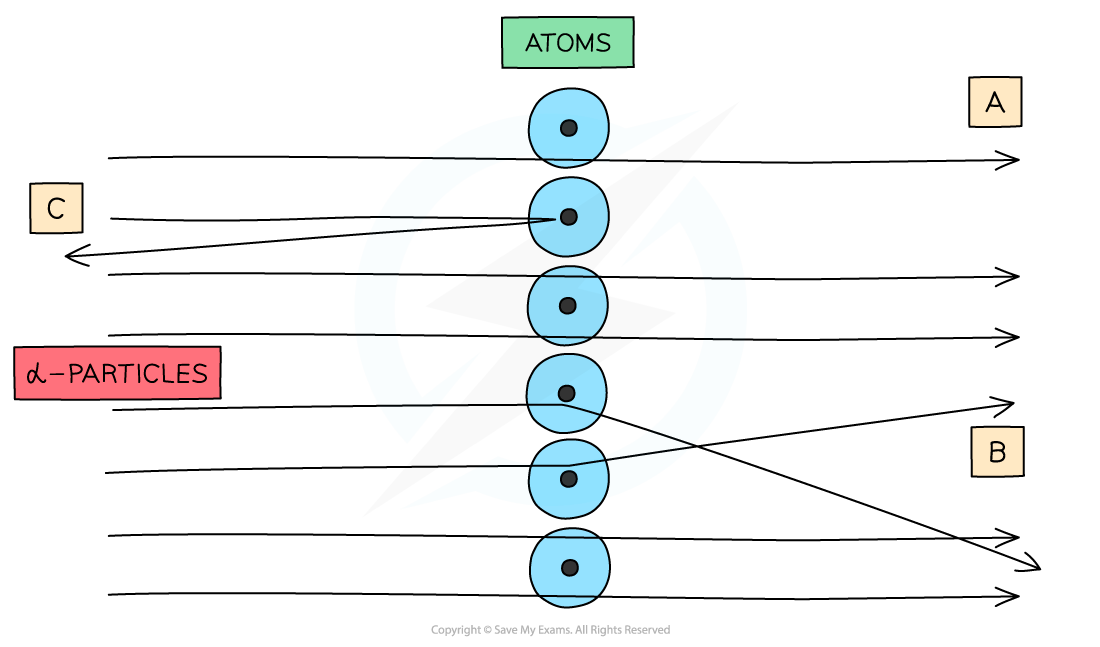When α-particles are fired at thin gold foil, most of them go straight through but a small number bounce straight back

• From this experiment, Rutherford results were:
• The majority of α-particles went straight through (A)
• This suggested the atom is mainly empty space
• Some α-particles deflected through small angles of < 10o (B)
• This suggested there is a positive nucleus at the centre (since two positive charges would repel)
• Only a small number of α-particles deflected straight back at angles of > 90o (C)
• This suggested the nucleus is extremely small and this is where the mass and charge of the atom is concentrated
• It was therefore concluded that atoms consist of small dense positively charged nuclei, surrounded by negatively charged electrons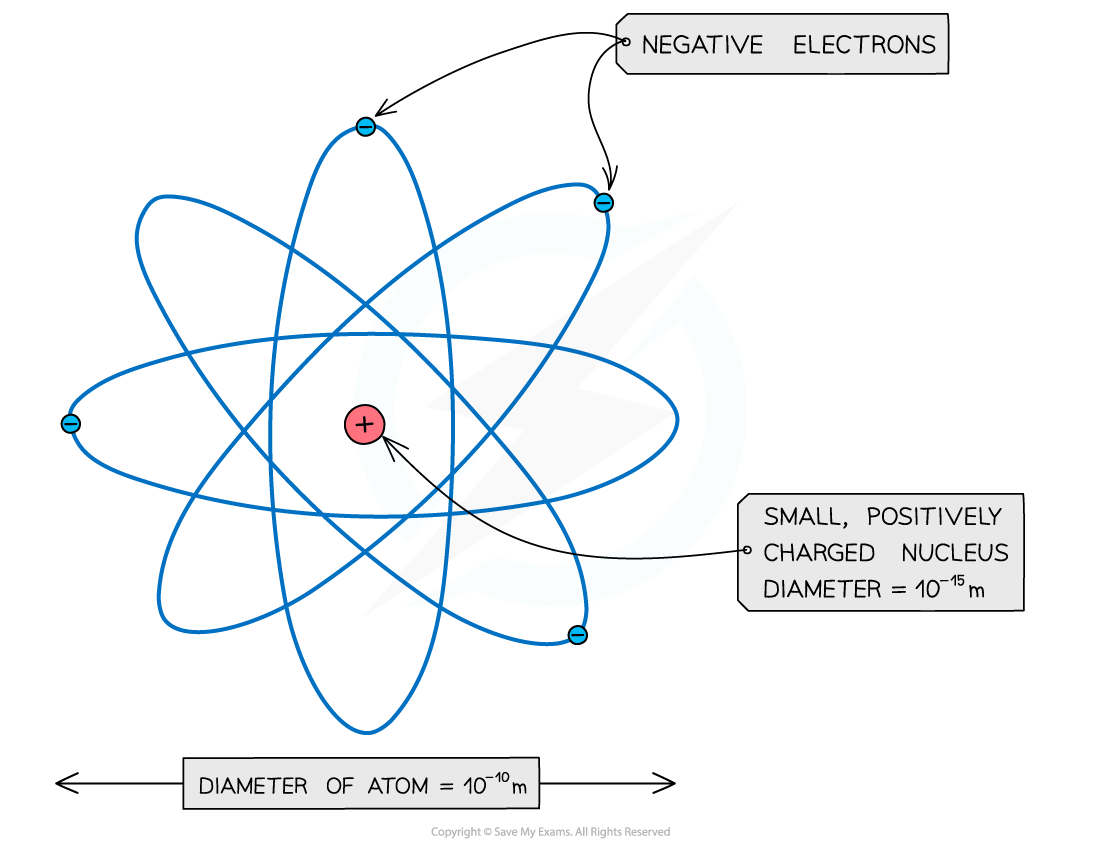An atom: a small positive nucleus, surrounded by negative electrons

• (Note: The atom is around 100,000 times larger than the nucleus!)

#### Worked Example

In an α-particle scattering experiment, a student set up the apparatus below to determine the number n of α-particle incident per unit time on a detector held at various angles θ.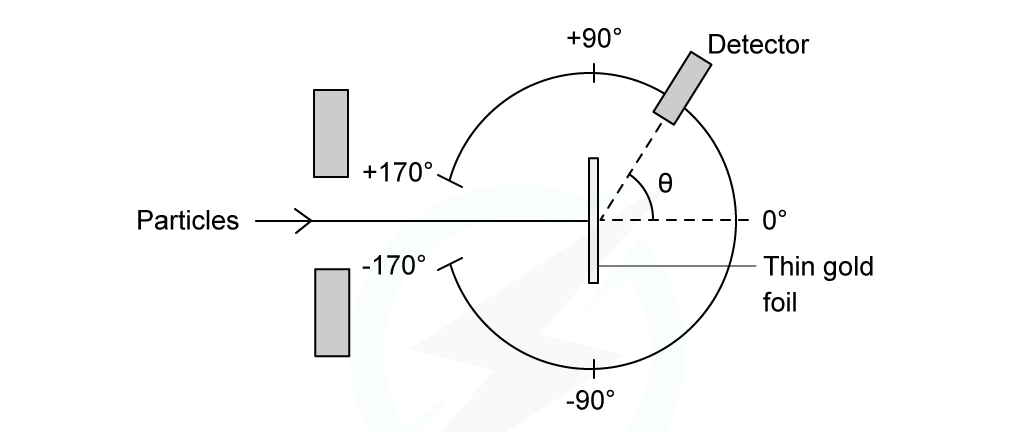Which of the following graphs best represents the variation of n with θ from 0 to 90°?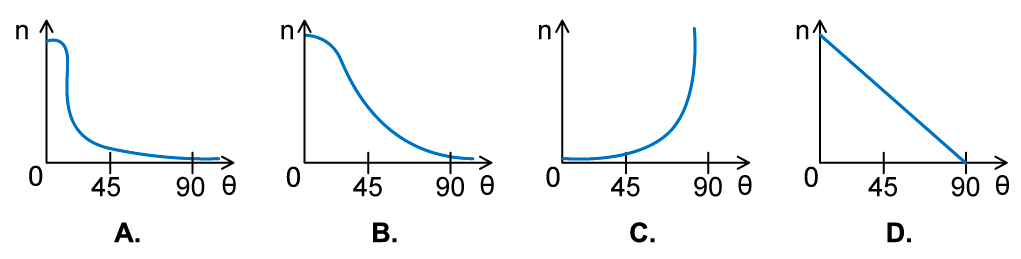• The Rutherford scattering experience directed parallel beams of α-particles at gold foil
• The observations were:
• Most of the α-particles went straight through the foil
• The largest value of n will therefore be at small angles
• Some of the α-particles were deflected through small angles
• n drops quickly with increasing angle of deflection θ
• These observations fit with graph A